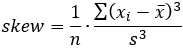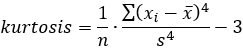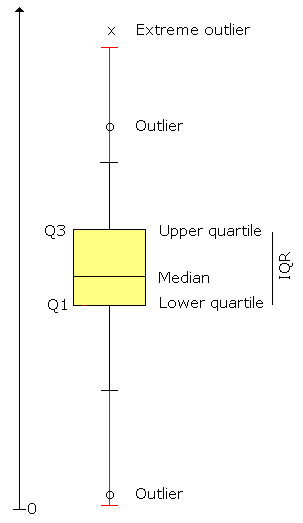# Metrics basics

Metrics are a tool for quality control and project management. They measure different attributes of a project or smaller pieces of code. For example, a metric may measure the number of code lines, the complexity of code or the amount of comments. Metrics can be used to find areas that are prone to problems. Metrics can be tracked across multiple teams or projects or they can be used to monitor the development of a single system.

What can you measure with code metrics? Code size is one of the first things to consider. Other metrics tell about the understandability and complexity of the code. There is more than one metric to evaluate for each of these properties.

It is to be noted that Project Metrics is about code-related metrics. We don't speak of money or schedule related metrics now. Here we are concerned about metrics that can be mechanically counted by reading the source code.

### Size

Code size is usually measured in lines of code or kilobytes. You can measure sizes such as project size, procedure size, source file size, executable file size and so on.

Lines of code

### Understandability

Some code is easy to read, other code is not. Low understandability will most probably result in more errors and maintaining problems.

Understandability metrics

### Complexity

High complexity in code may result in bad understandability and more errors. Complex code needs more time to develop, test and maintain. Therefore, excessive complexity should be avoided.

There are many kinds of software complexity.

• Structural complexity comes from conditional statements, loops, and the relationships between procedures, classes and files. Structural complexity tells about the control flow at run-time.
• Psychological complexity means how difficult it is to understand a program. This is very closely related to structural complexity.
• Informational complexity is about how data moves in a program. How much data goes into a procedure and how much comes out. It's a data flow issue.
• Mathematical/computational complexity is about how much time and memory it takes to execute an algorithm.

Code analysis finds metrics for structural and informational complexity. The following metrics are some of the best ones for evaluating the complexity of a project, file or a procedure: Lines of code, cyclomatic complexity, informational complexity, and structural fan-out.

Complexity metrics

### Object-oriented metrics

Metrics related to the object-oriented features of a program are often classified as object-oriented (OO) metrics. Historically, metrics were first developed for procedure-oriented (structured) code. Later, series of OO metrics were introduced. OO metrics are suitable for programs that consist of classes. It is to be noted that most if not all non-OO metrics are directly applicable to OO projects as well.

Object-oriented metrics

### Other metrics

There are other metrics which cannot be calculated mechanically by reading the source code. These include things like number of function points, money spent/1000 LOC, number of errors per module and time spent writing a program. You can combine code metrics with external data to calculate this kind of metrics by yourself.

### What are the target values?

There are no widely accepted target values for most of the metrics. This help gives some sample values for your consideration. You will have to make up your own goals. Take the Design quality report for some projects, and see how they differ. Then use the Metrics view feature (in the Enterprise menu) to list the procedures with the highest values, and see if something should be done. This way, you can learn to use metrics the way that suits you best.

## Statistics

This is a short introduction to the statistical values provided. If you need more information, consult any elementary statistics book.

Average, mean
Regular arithmetic average, sum/count.
Median
When you sort all the values in size order, median is the value that falls in the middle of the line. If there is an even number of values, median is the average of the two values in the middle.
5%..95% range, Q5..Q95
5% of the values are less than or equal to the Q5 value. 5% of the values are equal to or higher than the Q95 value. The rest 90% of values fall between these limits.
Range, min..max
Range tells you the minimum and maximum values. All other values are between these ones.
StdDev
Standard deviation, measurement of variation. Statistically, 2/3 of the values lie in the range [Average-StdDev, Average+StdDev].
Outliers
Outliers are high and low values that fall out of the range of typical values. The outliers are calculated statistically and they help you to spot code that may require a review or a rewrite.
Extreme outliers
Extreme outliers are similar to outliers, but they are defined with a higher upper limit and a smaller lower limit, so they are fewer than outliers. As extreme outliers are rare, they are a useful tool when there too many outliers at hand.
SkewSkewness is an advanced statistical indicator that characterizes the degree of asymmetry of a metric around its mean value. A lot of metrics have positive skew, which means the metric is skewed to the left. With negative skew the metric is skewed to the right.
KurtosisKurtosis is an advanced statistical indicator that characterizes the relative peakedness or flatness of a metric. Positive kurtosis indicates a relatively peaked distribution, which is typical to many metrics. Negative kurtosis indicates a relatively flat distribution.

#### OutliersOutliers are values that are either higher or lower than most other values. Outlier values are exceptional in the data at hand. You can watch for outliers to spot abnormal code that might require a closer review and possibly a rewrite. This works regardless of the metric in question (well, for most metrics anyway).

Being an outlier does not necessarily mean the code is bad. In fact, the code may be exceptionally good! An outlier value tells you that in some sense, the code is exceptional compared to other code in the same system. When you analyze another system you are likely to different outlier limits, and you're thus able to find the exceptional parts of that system.

How to define outlier limits? In a set of data, 1/4 of values fall in the low quartile, Q1 being the highest value. Respectively, 1/4 of values are in the high quartile, Q3 being the lowest value. The rest of the values (1/2 of all) lie between Q1 and Q3. The length of this range is called the inter-quartile range (IQR) and it is calculated as follows:

IQR = Q3 - Q1

We will then use the Q1, Q3 and IQR to define lower and upper limits of typical values in the data.

Upper limit = Q3 + 1.5 * IQR Lower limit = Q1 - 1.5 * IQR

Values below the lower limit are considered outliers. Similarly, values above the upper limit are considered outliers. The rest of the values are considered normal.

##### Extreme outliers

Extreme outliers are defined similarly to outliers, but using a higher upper limit and a smaller lower limit.

Extreme upper limit = Q3 + 3 * IQR Extreme lower limit = Q1 - 3 * IQR

Outliers or extreme outliers, which ones should you consider? If there are too many outliers at hand, you could first tackle the extreme ones. On the other hand, if there are few or no extreme outliers, watch out for the regular outliers (mild outliers, so to speak).

##### No outliers

It often happens that there is no lower limit (or no upper limit, or neither of them). In this case, all values at the low (high) end are considered normal. Even the minimum (maximum) value is relatively close to other values and therefore cannot be considered abnormal. In many cases, there are no outliers to find at all. In this case, all the code is relatively similar as far as that metric is concerned.

In the case of normally distributed data, only about 1 in 150 observations will be an outlier and only about 1 in 425,000 an extreme outlier. In the real world, software metrics are rarely normally distributed, so the amount of outliers and extreme outliers will vary.

##### How to work with outliers?

The Limits functionality lets Project Metrics automatically highlight outliers and extreme outliers in red to catch your attention. Select either the <Outliers> or the <Outliers, extreme> rule set to quickly apply outlier limits to all metrics.

Click the metric column header to sort the items by metric value. This way you can easily spot the red outliers.

Select the chart "Kiviat of limits on page" in the View menu. Together with the <Outliers> or the <Outliers, extreme> rule set, this is a quick way to spot metrics having a large or a small number of outliers.

©Aivosto Oy - Project Analyzer Help Contents